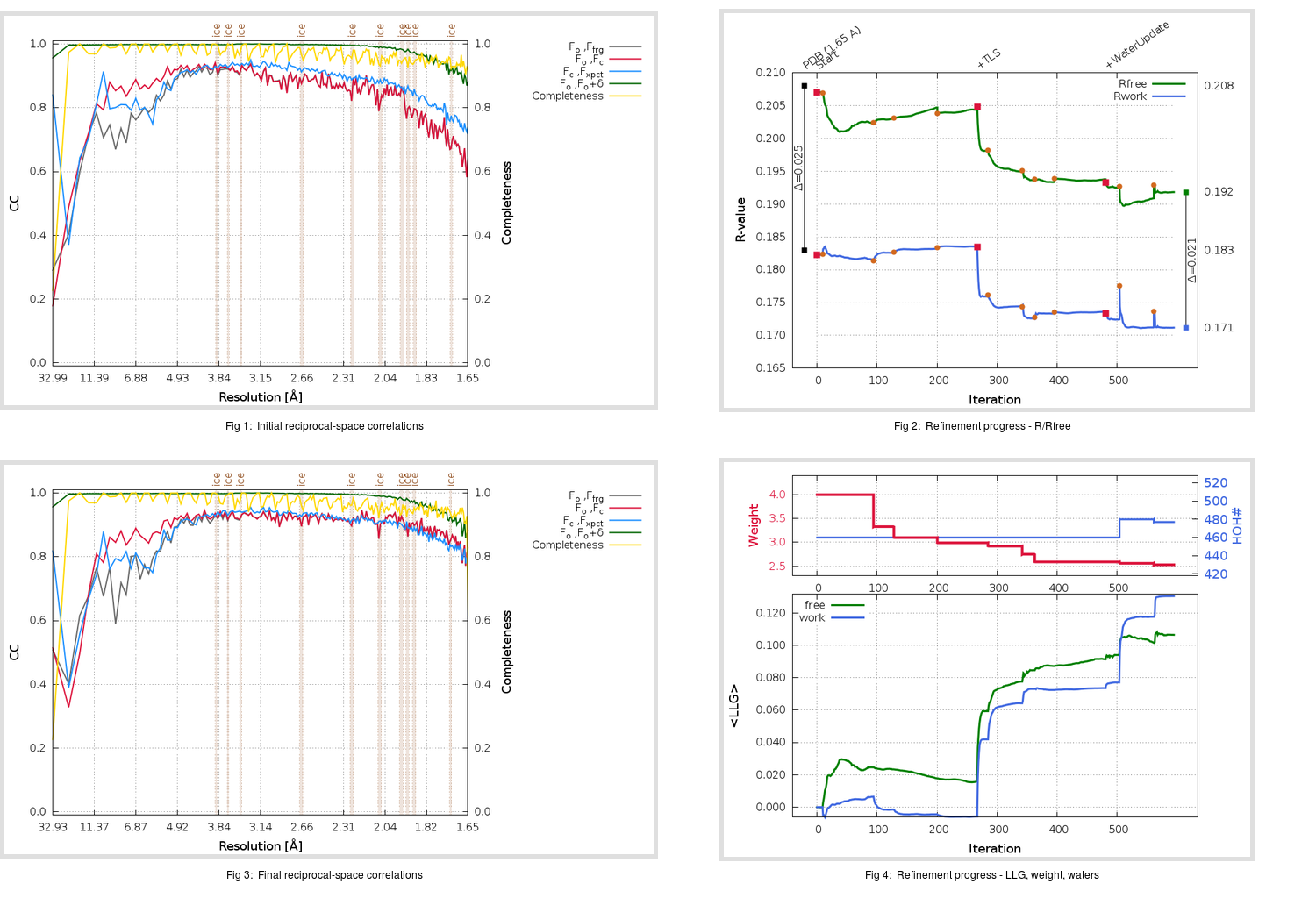Content:

```    Diffraction limits & principal axes of ellipsoid fitted to diffraction cut-off surface:
1.618         0.9652   0.0000   0.2614       a* + 0.006 c*
1.642         0.0000   1.0000   0.0000       b*
1.595        -0.2614   0.0000   0.9652      -0.470 a* + 0.882 c*
```

## Deposited

` `
 Date deposited Date data collection Resolution R, Rfree 20081012 20050320 1.65 0.1830 0.2080

Molprobity (CCP4 7.0 version) summary:

```Ramachandran outliers =   0.00 %
favored =  98.36 %
Rotamer outliers      =   2.66 %
C-beta deviations     =     1
Clashscore            =   7.10
RMS(bonds)            =   0.0201
RMS(angles)           =   1.99
MolProbity score      =   1.71
Resolution            =   1.65
R-work                =   0.1830
R-free                =   0.2080
```

```Number of waters      =   460

<B> (all atoms) =   26.47 ( sd =    9.74 ) for       2876 non-hydrogen atoms
<B>   (protein) =   23.97 ( sd =    7.40 ) for       2371 non-hydrogen atoms
<B>     (water) =   39.80 ( sd =    9.97 ) for        460 non-hydrogen atoms
<B>    (others) =   22.53 ( sd =    4.50 ) for         45 non-hydrogen atoms

B min/max       (all non-hydrogen atoms) =   11.67 /   78.31
B min/max   (protein non-hydrogen atoms) =   11.67 /   55.96
B min/max     (water non-hydrogen atoms) =   16.30 /   78.31
B min/max     (other non-hydrogen atoms) =   16.45 /   33.74
```

## BUSTER (re-)refinement

` `

Molprobity (CCP4 7.0 version) summary:

```Ramachandran outliers =   0.00 %
favored =  98.03 %
Rotamer outliers      =   2.28 %
C-beta deviations     =     1
Clashscore            =   1.46
RMS(bonds)            =   0.0112
RMS(angles)           =   1.57
MolProbity score      =   1.16
Resolution            =   1.65
R-work                =   0.1712
R-free                =   0.1918
```

```Number of waters      =   477

<B> (all atoms) =   27.33 ( sd =   11.32 ) for       2893 non-hydrogen atoms
<B>   (protein) =   24.24 ( sd =    7.71 ) for       2371 non-hydrogen atoms
<B>     (water) =   43.28 ( sd =   13.33 ) for        477 non-hydrogen atoms
<B>    (others) =   21.55 ( sd =    3.71 ) for         45 non-hydrogen atoms

B min/max       (all non-hydrogen atoms) =   13.30 /   76.97
B min/max   (protein non-hydrogen atoms) =   13.30 /   61.44
B min/max     (water non-hydrogen atoms) =   15.38 /   76.97
B min/max     (other non-hydrogen atoms) =   17.31 /   28.51
```

Refinement progression:Results:

` `
 File Remark 2ZU5_aB_refine.01_03_refine.pdb.gz exact refinement commands are in header 2ZU5_aB_refine.01_03_refine.mtz.gz including original deposited data and several re-refinement map coefficients 2ZU5_aB_refine.01_03_BUSTER_model.cif.gz including any non-standard compound restraints 2ZU5_aB_refine.01_03_BUSTER_refln.cif.gz